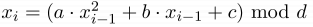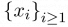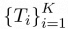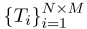## Day 2, Problem 2 - Random Number Generator

Little H has recently been studying randomized algorithms. Randomized algorithms often use random number generation functions (e.g. random from Pascal and rand from C/C++) to obtain their randomness. In reality, random number functions are not truly "random." Instead, they work off of some specific algorithms.

As such, the following recursive quadratic polynomial is one method:

The algorithm selects nonnegative integers x0, a, b, c, and d as its seed values and uses the following recursive calculations to generate a random number.

For any i ≥ 1,$\textstyle x_i = (a\cdot x^2_{i-1} + b\cdot x_{i-1}+c) \textup{ mod }d$

This way, a sequence of nonnegative integers$\textstyle \left \{ x_i \right \}_{i \ge 1}$ of arbitrary length can be obtained. Typically, we can consider this sequence to be random. Using the sequence$\textstyle \left \{ x_i \right \}_{i \ge 1}$, we can use the following algorithm to produce$\textstyle \left \{ T_i \right \}_{i=1}^K$, a random permutation of the numbers 1 to K.

1. Initialize T to the sequence of integers from 1 to K.
2. Perform K swaps on the sequence T. The i-th swap will swap the value of Ti with the value of T(xi mod i) + 1.

Outside of this base number of K swaps, little H has made an additional Q swaps. For the i-th additional swap, little H will choose two positions ui and vi and swap the values of Tui and Tvi.

To check the effectiveness of the random permutation generator, little H designed the following problem:

Little H has an N row by M column grid. She initially follows the above process, producing a random permutation$\textstyle \left \{ T_i \right \}_{i=1}^{N\times M}$ of the integers from 1 to N×M after N×M + Q swaps. Then these N×M values are then placed back into the grid, row for row, column for column. That is, the cell at column j of row i in the original grid will now take on the value of T(i−1)·M+j.

Afterwards, little H wishes to start from the top-left corner of the grid (i.e. row 1, column 1), each step moving either right or down under the precondition that she does not move outside of the grid, and reach the bottom-right corner (i.e. row N, column M).

Little H writes down the value of every cell she travels through, ordered from least to greatest. This way, for any valid path, little H can obtain an increasing sequence of length N + M − 1 which we will call the path sequence. Little H wishes to know the lexicographically smallest path sequence that she can obtain.

### Input Format

Line 1 of input consists of five integers x0, a, b, c, and d, representing the seed values to little H's random number generator.
Line 2 of input consists of three integers N, M, and Q, indicating that little H generates a permutation from 1 to N×M to fill her N×M grid. Also, after little H performs her N × M swaps, she will perform an additional Q swaps.
The final Q lines will each contain two integers ui and vi, indicating that the i-th additional swap involves swapping Tui and Tvi.

### Output Format

The output should consist of one line containing N + M − 1 space-separated positive integers, representing the lexicographically smallest path sequence that little H can obtain.

### Sample Input 1

1 3 5 1 71
3 4 3
1 7
9 9
4 9


### Sample Output 1

1 2 6 8 9 12


### Sample Input 2

654321 209 111 23 70000001
10 10 0


### Sample Output 2

1 3 7 10 14 15 16 21 23 30 44 52 55 70 72 88 94 95 97


### Sample Input 3

123456 137 701 101 10000007
20 20 0


### Sample Output 3

1 10 12 14 16 26 32 38 44 46 61 81 84 101 126 128 135 140 152 156 201 206 237 242 243 253 259 269 278 279 291 298 338 345 352 354 383 395


### Explanation

For sample 1, according to the input seed values, the first 12 random numbers of xi are:
9 5 30 11 64 42 36 22 1 9 5 30
With these 12 random numbers, little H will perform 12 swap operations, yielding the following:
6 9 1 4 5 11 12 2 7 10 3 8
After the additional 3 swap operations, little H obtains the final permuted sequence of:
12 9 1 7 5 11 6 2 4 10 3 8
This sequence will yield the following grid.

 12 9 1 7 5 11 6 2 4 10 3 8

The optimal path sequence is: 12→9→1→6→2→8.

### Constraints

The constraints of all the test cases are outlined below.

Test CaseN, MQOther Constraints
12 ≤ N, M ≤ 8 Q = 0 0 ≤ a ≤ 300
0 ≤ b, c ≤ 108
0 ≤ x0 < d ≤ 108
1 ≤ ui, viN × M
22 ≤ N, M ≤ 200
3
42 ≤ N, M ≤ 20000 ≤ Q ≤ 50000
5
6
72 ≤ N, M ≤ 5000
8
9
10

### Warning

This problem's memory limit is 256MB. Please ensure that the total memory of the execution of your submitted source code does not exceed this limit.
A 32-bit integer (e.g. int from C/C++ and Longint from Pascal) is four bytes long. So if your program declares a size 1024×1024 array of 32-bit integers, 4MB of memory will be used.

Point Value: 20 (partial)
Time Limit: 5.00s
Memory Limit: 256M